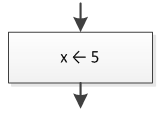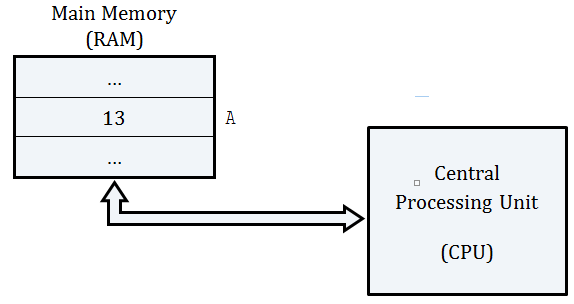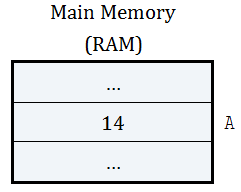The most commonly used operator in a computer language is the value assignment operator ( = ). For example, the statement

\$x = 5;

x = 5;

## Visual Basic, Python

x = 5

assigns a value of 5 to variable x.

As you already know, this is equivalent to the left arrow used in flowcharts.Probably the left arrow used in a flowchart is more convenient and clearer than the value assignment operator ( = ) sign because it shows in a more graphical way that the value or the result of an expression on the right is assigned to a variable on the left.

Be careful! The ( = ) sign is not equivalent to the one used in mathematics. In mathematics, the expression` x = 5` is read as “x is equal to 5. However, in a computer language the expression` x = 5` is read as “assign the value 5 to x” or “set x equal to 5.” They look the same but they act differently!

In mathematics, the following two lines are equivalent:

`x = y + z`

`y + z = x`

The first one can be read as “x is equal to the sum of y and z” and the second one as “the sum of y and z is equal to x.”

On the other hand, in a computer language, the following two statements are definitely not equivalent. In fact, the second one is considered wrong!

\$x = \$y + \$z;
\$y + \$z = \$z;

x = y + z;
y + z = x;

## Visual Basic, Python

x = y + z
y + z = x

The first statement seems quite correct. It can be read as “Set x equal to the sum of y and z” or “Assign the sum of y and z to x.

But what about the second one? Think! Is it possible to assign the value of x to y + z? The answer is obviously a big “NO!”

Remember! In a computer language, the variable on the left side of the ( = ) sign represents a region in main memory (RAM) where a value can be stored. Thus, on the left side only one single variable must exist! However, on the right side there can be a number, a variable, a string, or even a complex mathematical expression.

In Table 1 you can find some examples of value assignments.

## PHP

 Table 1 Examples of Value Assignments `\$a = 9;` Assign a value of 9 to variable` a`. `\$b = \$c;` Assign the content of variable` c` to variable` b`. `\$d = "Hello Zeus";` Assign the string Hello Zeus to variable` d`. `\$d = \$a + \$b;` Calculate the sum of the contents of variables` a` and` b` and assign the result to variable` d`. `\$b = \$a + 1;` Calculate the sum of the content of variable` a` and 1 and assign the result to variable` b`. Please note that the content of variable` a` is not altered. `\$a = \$a + 1;` Calculate the sum of the content of variable` a `and 1 and assign the result back to variable` a`. In other words, increase variable` a `by one.

## Java, C++, C#

 Table 1 Examples of Value Assignments `a = 9;` Assign a value of 9 to variable` a`. `b = c;` Assign the content of variable` c` to variable` b`. `d = "Hello Zeus";` Assign the string Hello Zeus to variable` d`. `d = a + b;` Calculate the sum of the contents of variables` a` and` b` and assign the result to variable` d`. `b = a + 1;` Calculate the sum of the content of variable` a` and 1 and assign the result to variable` b`. Please note that the content of variable` a` is not altered. `a = a + 1;` Calculate the sum of the content of variable` a `and 1 and assign the result back to variable` a`. In other words, increase variable` a `by one.

## Visual Basic, Python

 Table 1 Examples of Value Assignments `a = 9` Assign a value of 9 to variable` a`. `b = c` Assign the content of variable` c` to variable` b`. `d = "Hello Zeus"` Assign the string Hello Zeus to variable` d`. `d = a + b` Calculate the sum of the contents of variables` a` and` b` and assign the result to variable` d`. `b = a + 1` Calculate the sum of the content of variable` a` and 1 and assign the result to variable` b`. Please note that the content of variable` a` is not altered. `a = a + 1` Calculate the sum of the content of variable` a `and 1 and assign the result back to variable` a`. In other words, increase variable` a `by one.

Confused about the last one? Are you thinking about your math teacher right now? What would he/she say if you had written` a = a + 1` on the blackboard? Can you personally think of a number that is equal to the number itself plus one? Are you nuts? This means that 5 is equal to 6 and 10 is equal to 11!

Obviously, things are different in computer science. The statement` a = a + 1` is absolutely acceptable! It instructs the CPU to retrieve the value of variable` a` from main memory (RAM), to increase the value by one, and to assign the result back to variable` a`. The old value of variable` a` is replaced by the new one.

Still don’t get it? Let’s take a look at how the CPU and main memory (RAM) cooperate with each other in order to execute the statement` A ← A + 1`.

Let’s say that there is a region in memory, named` A` and it contains the number 13When a program instructs the CPU to execute the statement

`A ← A + 1`

the following procedure is executed:

• the number 13 is transferred from the RAM’s region named` A` to the CPU;
• the CPU calculates the sum of 13 and 1; and
• the result, 14, is transferred from the CPU to the RAM’s region named` A` replacing the existing number, 13.

After execution, the RAM looks like this.